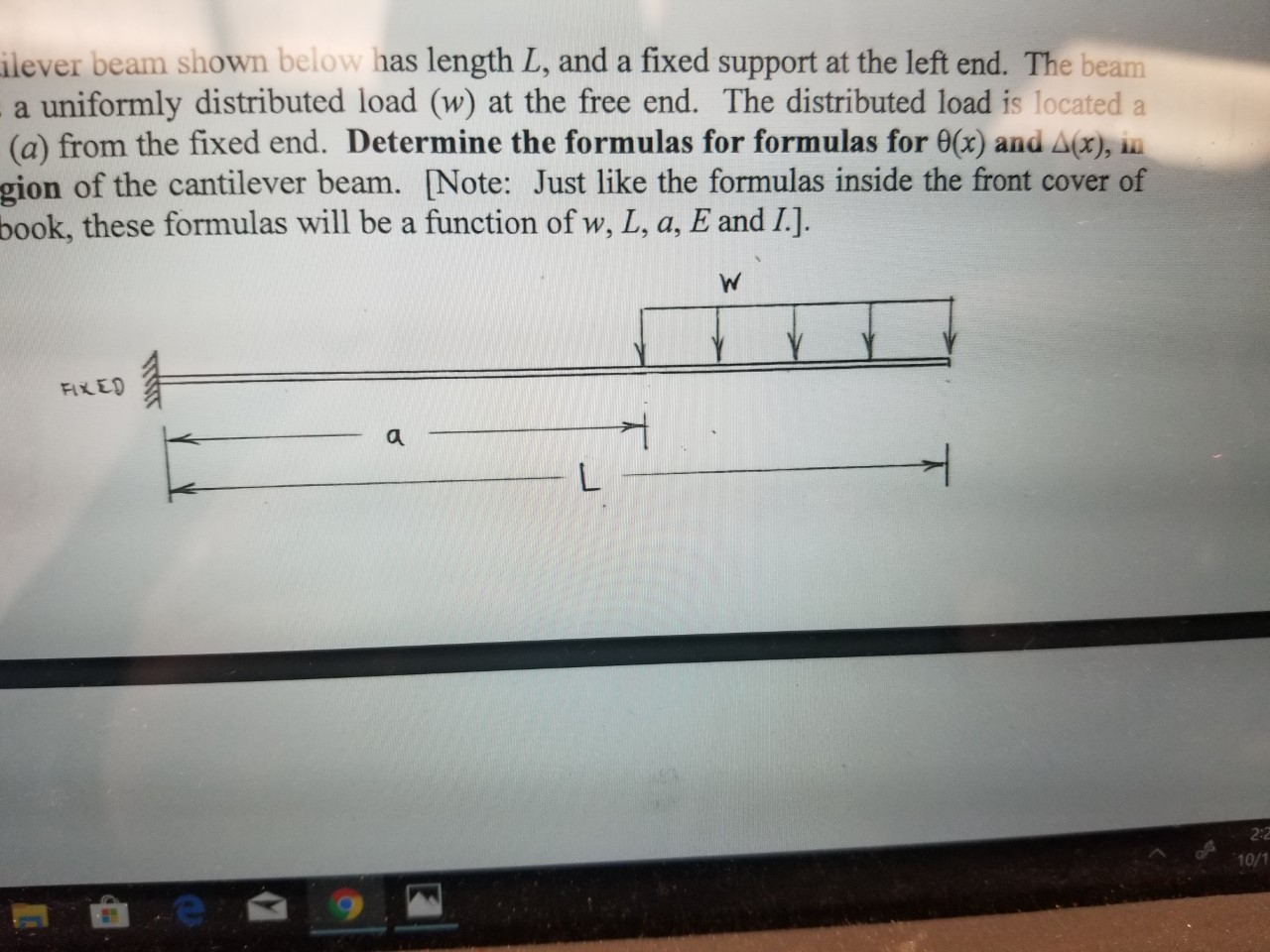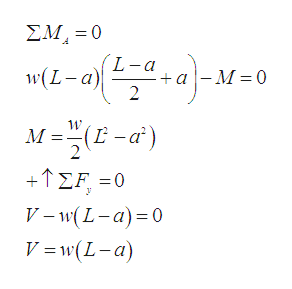# ilever beam shown below has length L, and a fixed support at the left end. The beama uniformly distributed load (w) at the free end. The distributed load is located a(a) from the fixed end. Determine the formulas for formulas for 0x) and Ax), ingion of the cantilever beam. [Note: Just like the formulas inside the front cover ofbook, these formulas will be a function of w, L, a, E and I.].WFIXED2:210/1

Question
9 views

Using the double integration method, determine the formulas for slope and deflection in each region of the beam.help_outlineImage Transcriptioncloseilever beam shown below has length L, and a fixed support at the left end. The beam a uniformly distributed load (w) at the free end. The distributed load is located a (a) from the fixed end. Determine the formulas for formulas for 0x) and Ax), in gion of the cantilever beam. [Note: Just like the formulas inside the front cover of book, these formulas will be a function of w, L, a, E and I.]. W FIXED 2:2 10/1 fullscreen
check_circle

Step 1
Step 2

Consider the cantilever beam as ABC.

Sketch the Free Body Diagram as shown below.

Step 3

Refer to Figure 1.

Use equil...help_outlineImage TranscriptioncloseΣΜ-0 L-a w(L-a) "e-3Nο) - M 0 2 M=F E -α) Μ: + ΣF-0 V -w(L-a)0 V =w(L-a) fullscreen

### Want to see the full answer?

See Solution

#### Want to see this answer and more?

Solutions are written by subject experts who are available 24/7. Questions are typically answered within 1 hour.*

See Solution
*Response times may vary by subject and question.
Tagged in